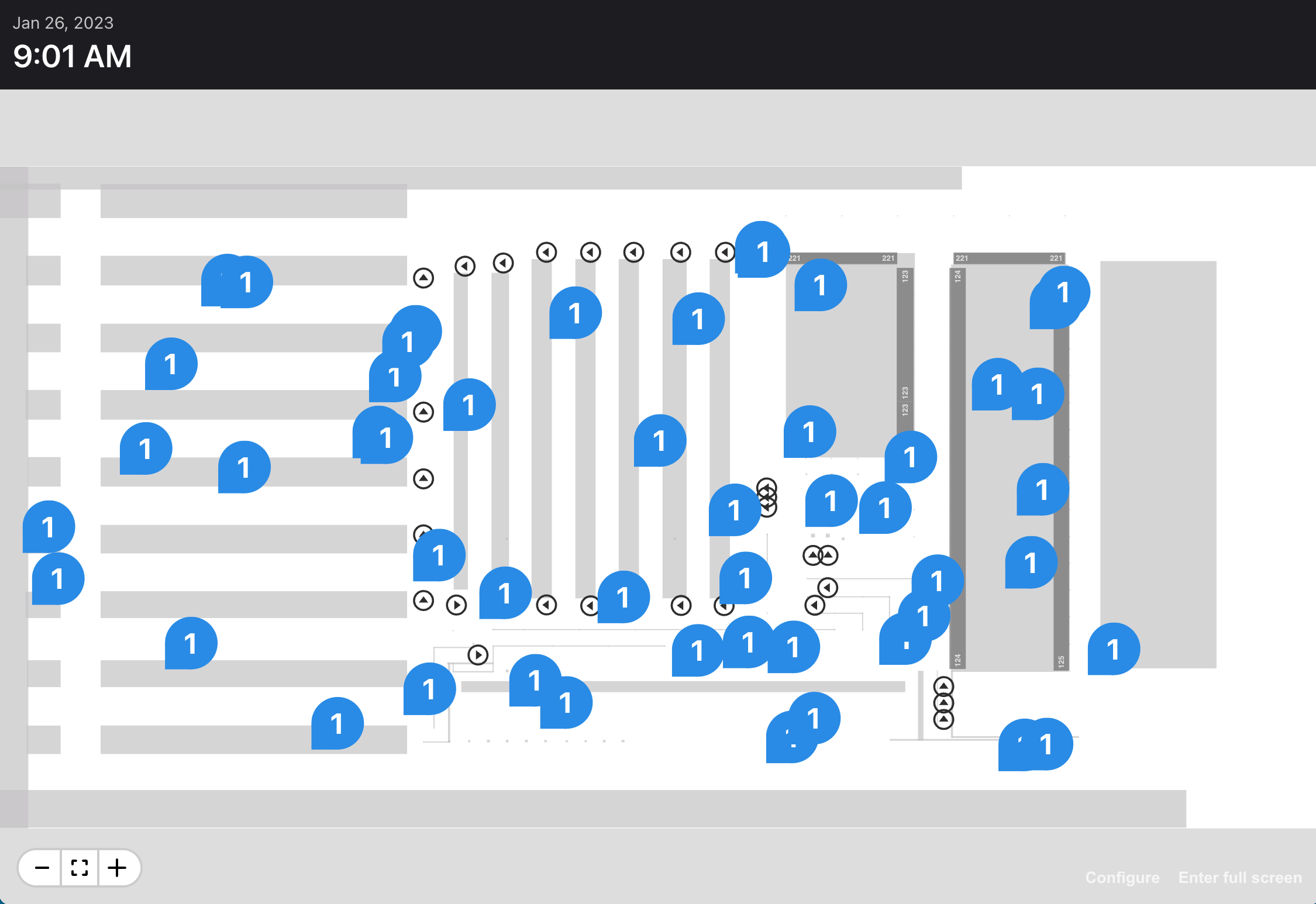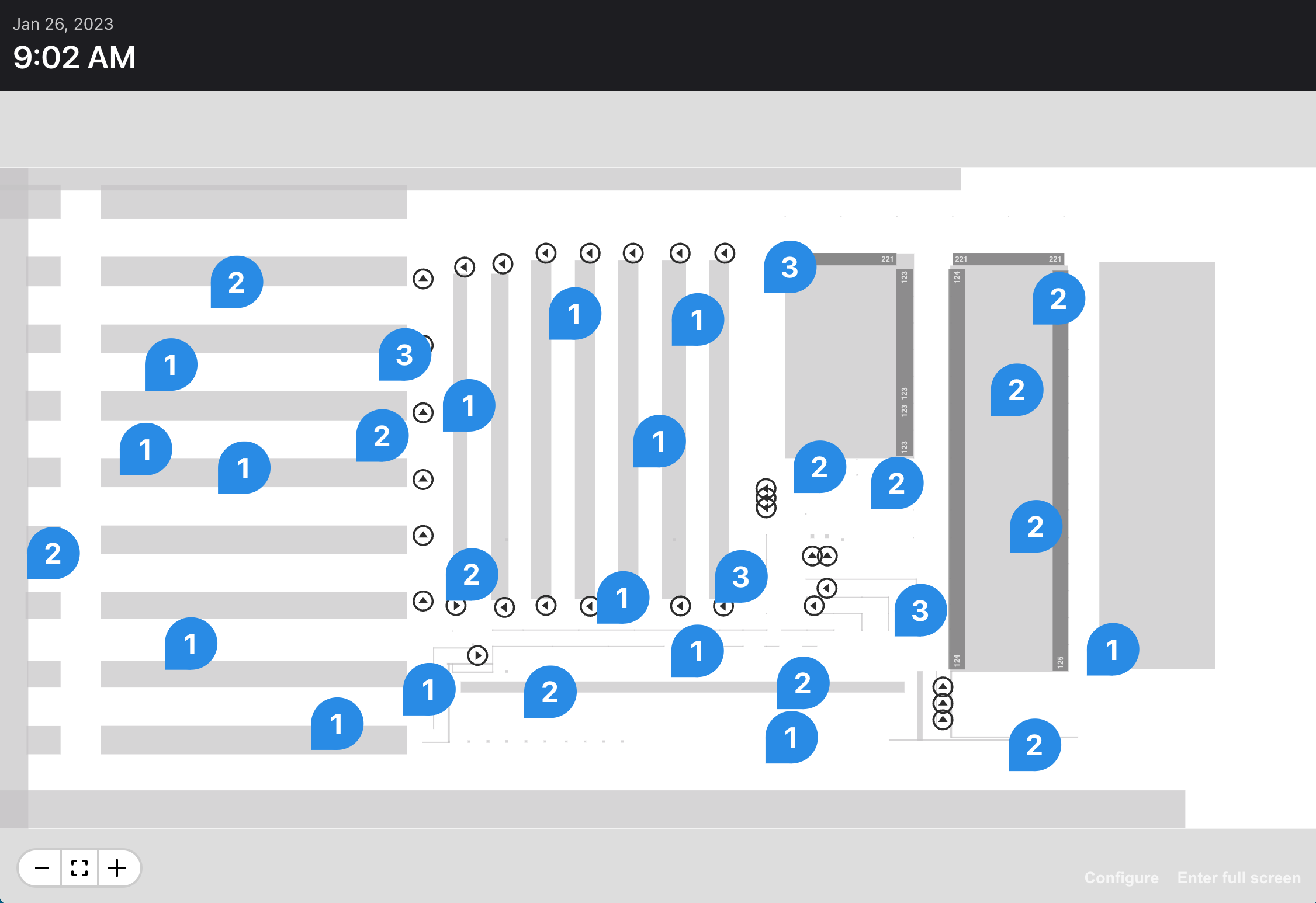Home

# Clustering markers on a warehouse map

### Background

From 2020 to 2023, I developed dashboards and mapping tools for a warehouse automation system using TypeScript, React, SVG and Canvas.

### Problem

Our custom mapping system supported the arbitrary placement of "markers." Sometimes these markers would overlap, obscuring their values, as shown below. Our map developers needed a way to eliminate this clutter.### Solution

I created a simple clustering function that takes a set of inputs, each having a coordinate and count, and returns a smaller set of outputs, each having a coordinate and count. Then I could render exactly one marker per output:Here's the effect:

### The code

First, I created an Input type to represent a unit of data on the map:

``````type Input = {
key: string;
point: Point;
count: number;
};```
```

Then I created an Output type, which takes the same shape as an Input yet also retains a reference to its constituent inputs:

``````type Output = Input & {
inputs: Input[];
};```
```

Then I created a utility function that accepts an array of inputs, the current scale of the map, and a search radius (a larger radius packs more data). It returns a smaller array of outputs:

``````function cluster(inputs: Input[], scale: number, radius: number): Output[] {
...
return outputs;
}```
```

Finally, with just a few lines of code, I was able to eliminate the clutter in a data-intensive map. Below, the map displays one "pin" per output:

``````function DemoMap() {
...

const [scale, setScale] = useState(1);
const pinSize = 5;
const radius = pinSize * 1.5;
const inputs: Input[] = data;
const outputs: Output[] = cluster(inputs, scale, radius);

const pins = outputs.map((output) => {
return (
<Annotation key={output.key} x={output.point.x} y={output.point.y} scale="ignore">
<Pin color={Color.Blue} value={output.count} size={pinSize} />
</Annotation>
);
});

return (
<Map onZoom={(evt) => setScale(evt.scale)} ...>
<g>{pins}</g>
</Map>
);
}```
```

### Conclusion

My utility function was a straightforward solution to a sticky problem. In the next version, I'd like to support different types of inputs at the same time.